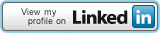## Connect Me## Pages

### QBASIC Programs: Factorial of a Number

REM QBASIC Program to get the factorial of a supplied number
DECLARE FUNCTION fact (n)
CLS
INPUT "Supply a number"; n
PRINT "Factorial value of"; n; "is"; fact(n)
END

FUNCTION fact (n)
f = 1
c = 1
WHILE c <= n f = f * c c = c + 1 WEND fact = f END FUNCTION ***********************************************
Ą